The OpenFOAM Foundation
NVDTVD Class Reference

## Public Types

typedef scalar phiType

## Public Member Functions

NVDTVD ()

scalar phict (const scalar faceFlux, const scalar phiP, const scalar phiN, const vector &gradcP, const vector &gradcN, const vector &d) const

scalar r (const scalar faceFlux, const scalar phiP, const scalar phiN, const vector &gradcP, const vector &gradcN, const vector &d) const

## Detailed Description

Definition at line 43 of file NVDTVD.H.

## Member Typedef Documentation

 typedef scalar phiType

Definition at line 48 of file NVDTVD.H.

Definition at line 49 of file NVDTVD.H.

## Constructor & Destructor Documentation

 NVDTVD ( )
inline

Definition at line 54 of file NVDTVD.H.

References NVDTVD::phict().

Here is the call graph for this function: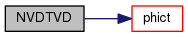## Member Function Documentation

 scalar phict ( const scalar faceFlux, const scalar phiP, const scalar phiN, const vector & gradcP, const vector & gradcN, const vector & d ) const
inline

Definition at line 61 of file NVDTVD.H.

References Foam::mag(), NVDTVD::r(), and Foam::sign().

Referenced by NVDTVD::NVDTVD().

Here is the call graph for this function: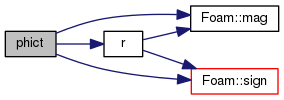Here is the caller graph for this function: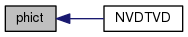scalar r ( const scalar faceFlux, const scalar phiP, const scalar phiN, const vector & gradcP, const vector & gradcN, const vector & d ) const
inline

Definition at line 95 of file NVDTVD.H.

References Foam::mag(), and Foam::sign().

Referenced by NVDTVD::phict().

Here is the call graph for this function: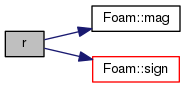Here is the caller graph for this function: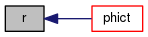The documentation for this class was generated from the following file:
• src/finiteVolume/interpolation/surfaceInterpolation/limitedSchemes/LimitedScheme/NVDTVD.H